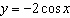Name:    MHF4U - 5.3 Equations of Sinusoidal

Multiple Choice
Identify the choice that best completes the statement or answers the question.

1.

Which graph of the following functions does not have vertical asymptotes?
 a. y =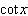c. y =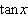b. y =d. y =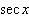2.

The graph of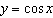has a period of
 a.c.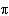b. 1 d.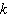3.

Determine an equation for the sinusoidal function shown.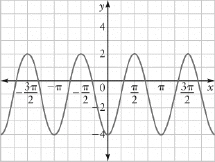a.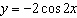c.b.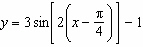d.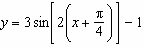4.

Determine an equation for the sinusoidal function shown.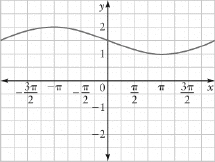a.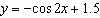c.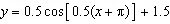b.d.5.

Determine an equation for the sinusoidal function shown.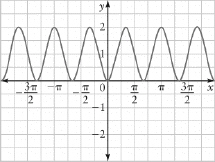a.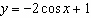c.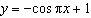b.d.